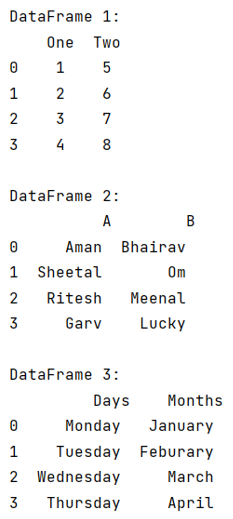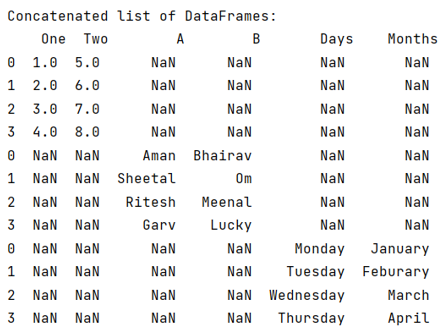# How to concatenate a list of pandas DataFrames together?

Given a list of Pandas DataFrames, we have to concatenate them.
Submitted by Pranit Sharma, on June 04, 2022

Pandas is a special tool which allows us to perform complex manipulations of data effectively and efficiently. Inside pandas, we mostly deal with a dataset in the form of DataFrame. DataFrames are 2-dimensional data structure in pandas. DataFrames consists of rows, columns and the data.

Here, we are going to learn how to concatenate the list of DataFrames, for this purpose we will first create multiple DataFrames, then we will create a list of multiple DataFrames and finally we will use pandas.concat() method and pass a list of DataFrames inside this method.

Syntax:

```pandas.concat(
objs,
axis=0,
join='outer',
ignore_index=False,
keys=None,
levels=None,
names=None,
verify_integrity=False,
sort=False,
copy=True
)
```

To work with pandas, we need to import pandas package first, below is the syntax:

```import pandas as pd
```

Let us understand with the help of an example,

```# Importing pandas package
import pandas as pd

# Creating three diffrent dictionaries
d1 = {
'One':[1,2,3,4],
'Two':[5,6,7,8]
}

d2 = {
'A':['Aman','Sheetal','Ritesh','Garv'],
'B':['Bhairav','Om','Meenal','Lucky']
}

d3 = {
'Days':['Monday','Tuesday','Wednesday','Thursday'],
'Months':['January','Feburary','March','April']
}

# Creating 3 DataFrames
df1 = pd.DataFrame(d1)
df2 = pd.DataFrame(d2)
df3 = pd.DataFrame(d3)

# Display all the DataFrames
print("DataFrame 1:\n",df1,"\n")
print("DataFrame 2:\n",df2,"\n")
print("DataFrame 3:\n",df3,"\n")
```

Output:Now, insert all these DataFrames into a list and use pandas.concat() method.

```# Inserting all the DataFrames into
# one single list
list = [df1,df2,df3]

# Concatenating the list of DataFrames
result = pd.concat(list)

# Display result
print("Concatenated list of DataFrames:\n",result)
```

Output:Preparation

What's New

Top Interview Coding Problems/Challenges!